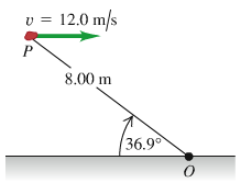# Problem: A 2.00-kg rock has a horizontal velocity of magnitude 12.0 m/s when it is at point P in the figure (Figure 1). At this instant, what are the magnitude and direction of its angular momentum relative to point O?

###### FREE Expert Solution

Angular momentum:

$\overline{){\mathbf{L}}{\mathbf{=}}{\mathbf{m}}{\mathbf{v}}{\mathbf{r}}{\mathbf{·}}{\mathbf{s}}{\mathbf{i}}{\mathbf{n}}{\mathbf{\theta }}}$

The angle between v and r is

θ = 180 - 36.9 = 143.1°

82% (486 ratings)###### Problem Details

A 2.00-kg rock has a horizontal velocity of magnitude 12.0 m/s when it is at point P in the figure (Figure 1).At this instant, what are the magnitude and direction of its angular momentum relative to point O?